• Call Now

1800-102-2727•

# Pseudo Force: Definition, Formula, Examples, Effects

Consider that you are travelling by bus and the bus starts accelerating forward. You will feel a backward force being applied on you by someone. This imaginary force that you felt is called pseudo force. We will study about Pseudo force and when this force comes into picture in detail. We will also see the magnitude and direction of pseudo forces.

• Difference between inertial and non inertial reference frame
• Pseudo force
• Apparent weight in lift
• Practice problems
• FAQs

## Pseudo force

When the weighing machine is kept inside a lift and the lift moves upwards, the reading shown will be more than the actual weight. When the lift moves downwards, the reading shown will be less than the actual weight. Can this be an example of violation of Newton's law?

Actually, Newton's laws are valid when the observer is in an inertial frame of reference.

For observers where Newton's laws are not valid, the frame is known as a non-inertial frame of reference. So to analyse the motion in a non-inertial frame we apply pseudo force.

The pseudo force is an imaginary force that is applied to objects in a non-inertial frame of reference to satisfy Newton’s laws of motion.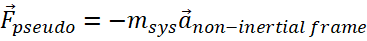Negative direction implies that the direction of force is opposite to acceleration of the system.

• It acts opposite to the direction of acceleration of the reference frame.
• It is applied to the non-inertial frame of reference.
• It is a fictitious force so there is no action-reaction pair.
• Pseudo force is also known as Inertial Force, although their need arises because of the use of non-inertial frames.

## Apparent Weight In Lift

Consider a block of mass m kept on a weighing machine. Consider the system is kept in an elevator. Let observer O1 observe this system from the ground frame. Let another observer O2 be present inside the lift.Case1: Lift moving upward with acceleration ‘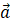With respect to O1

This observer finds the system is moving upwards with acceleration a. Other forces acting on

the block is its weight and normal reaction.Along the vertical direction,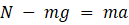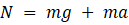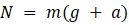As the weighing machine measures the normal reaction acting on it, the weighing machine will show a reading corresponding to..

With respect to O2

When the observer is in the elevator, the block is at rest with respect to him. So for this case,

The forces acting on the block are weight (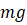) and normal reaction (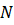).Along the vertical direction,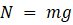This is completely wrong as we must get the same reading on the weighing machine. We got the wrong answer because O2 is in a non-inertial frame so Newton’s laws are not valid. To get the correct answer let’s apply pseudo force. In this case direction of pseudo force will be downward and magnitude is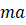. So here is the correct FBD by applying pseudo force:Along the vertical direction,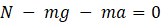So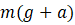is the apparent weight of a block of mass m in the lift accelerating upward so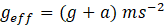Case2: Lift moving downward with acceleration ‘With respect to O1

This observer finds the system is moving downwards with acceleration a. Other forces acting on

the block is its weight and normal reaction.Along the vertical direction,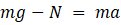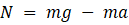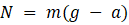As the weighing machine measures the normal reaction acting on it, the weighing machine will show a reading corresponding to.

With respect to O2

When the observer is in the elevator, the block is at rest with respect to him. So for this case,

The forces acting on the block are weight () and normal reaction ().Along the vertical direction,This is completely wrong as we must get the same reading on the weighing machine. We got the wrong answer because O2 is in a non-inertial frame so Newton’s laws are not valid. To get the correct answer let’s apply pseudo force. In this case direction of pseudo force will be upward and magnitude is. So here is the correct FBD by applying pseudo force:Along the vertical direction,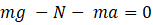So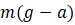is the apparent weight of a block of mass m in the lift accelerating downward so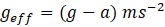.

Video Explanation: Pseudo Force (Time Stamp: 1.22.20 to 1.36.30)

## Practice problems

Question.1 A man weighs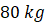. He stands on a weighing machine in a lift that is moving upwards with a uniform acceleration of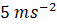. What would be the reading on the machine? (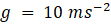)

Answer: Here, the observer will feel that he is at rest with respect to the lift as he has the same acceleration as that of the lift. Observer is in a non-inertial frame. Hence, pseudo force should be considered in this case.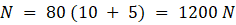Question.2 Figure shows a pendulum suspended from the roof of a truck that has a constant acceleration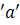towards the right relative to the ground. Find the deflection of the pendulum from the vertical.If we solve the problem in the truck frame, Let the observer be in the truck.

We have to apply pseudo force in the left direction on the bob as shown in FBD,Balancing forces in a horizontal direction,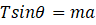……..(1)

Balancing forces in vertical direction,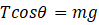……..(2)

Divide equation (1) and (2),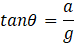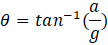Question.3 A block of mass m resting on a wedge of angle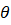as shown in the figure. The wedge is given an acceleration. What is the minimum value of a so that the mass m falls freely?Answer: For the block to fall freely, there should not be any normal reaction between wedge and block i.e.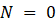.

Let’s solve it in a non-inertial (wedge) frame, So we have to apply pseudo force in the right direction as shown in the FBD,Now balance the forces in the Y direction,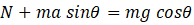Butthen,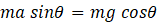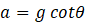Question.4 The accelerations of a particle as seen from two frames S1 and S2 have equal magnitude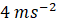. Then comment on the relationship between the acceleration of S1 and S2?Acceleration of particle P with respect to S1 is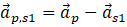Acceleration of particle P with respect to S2 is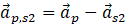Subtract above equations,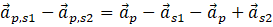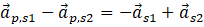By rearranging,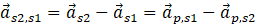So we can write,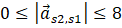So the acceleration of S2 with respect to S1 can be anything between zero and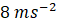.

## FAQs

Question.1 What is pseudo force?

Answer: The pseudo force is an imaginary force that is applied to objects in a non-inertial frame of reference to satisfy Newton’s laws of motion.

Question.2 What is the magnitude of pseudo force?

Answer: The magnitude of the pseudo force is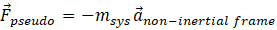.

Question.3 Is pseudo force necessary to apply in a non-inertial frame?

Answer: If you want to apply Newton’s laws of motion from a non-inertial frame then it is necessary to apply pseudo force as Newton’s laws are not valid in a non-inertial frame of reference.

Question.4 What is the direction of pseudo force?

Answer: Direction of pseudo force is opposite to the direction of acceleration of a non-inertial frame.

Related TopicsTalk to our expert
Resend OTP Timer =
By submitting up, I agree to receive all the Whatsapp communication on my registered number and Aakash terms and conditions and privacy policy